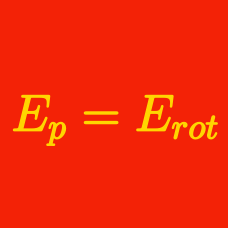Classical Mechanics

# Conservation of Energy: Level 2 Challenges

A light body and a heavy body have the same momentum.

Which of the two bodies will have greater kinetic energy?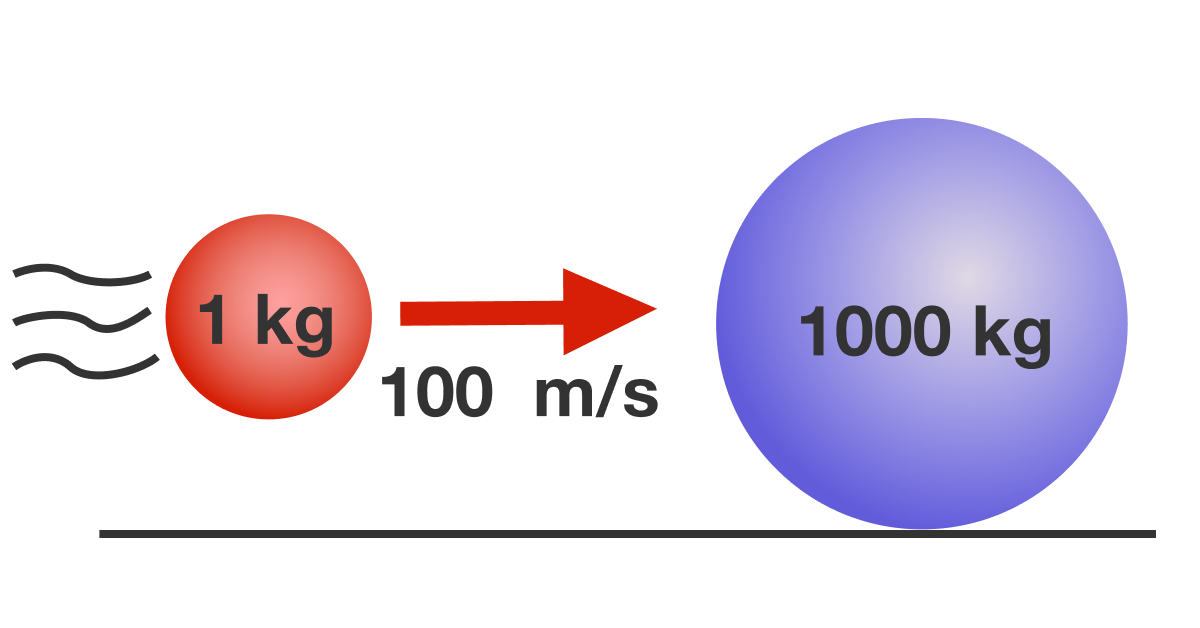A sphere of mass 1 kg is traveling with velocity 100 m/s toward a stationary sphere of mass 1000 kg. The two spheres collide elastically. What is the total kinetic energy (in Joules) of the spheres after the collision?

Assume that both spheres have the same radius. All surfaces are smooth. There is no rolling motion.

A pendulum with string of length 0.1 m is raised to an angle of $30^\circ$ below the horizontal, as shown below, and then released. What is the velocity (in m/s) of the pendulum when it reaches the bottom ?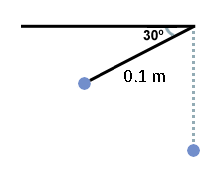Use: $g=10 \text{ m/s}^2$.

This is a problem of Energy Tranfers .

Scenario A: Bob attaches a mass $m$ at the end of a long string (length $L$) and holds the other end of the string, allowing the mass to swing like a pendulum, with amplitude $A$. When the mass reaches a turning point, Bob quickly pulls on the string so that the swinging part of the string shortens to $\tfrac12 L$. The pendulum now swings with a higher frequency.

Scenario B: Bob repeats the exact same situation: a pendulum length $L$ and mass $m$ is swinging with amplitude $A$. This time, Bob quickly pulls on the string when the mass passes through the equilibrium position. Again, the string is shortened to $\tfrac12 L$, and the pendulum swings with a higher frequency.

In which scenario, if any, will the pendulum have a greater amplitude after Bob shortens the string?

Assumptions: The size of the swinging mass is negligible. When Bob pulls on the string, he does this in a negligible amount of time. During the pull, the force is directed upward along the string.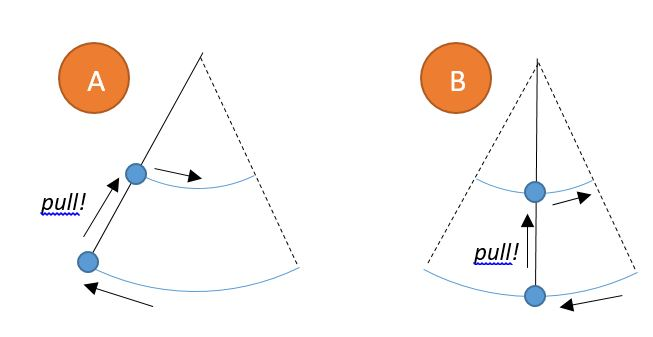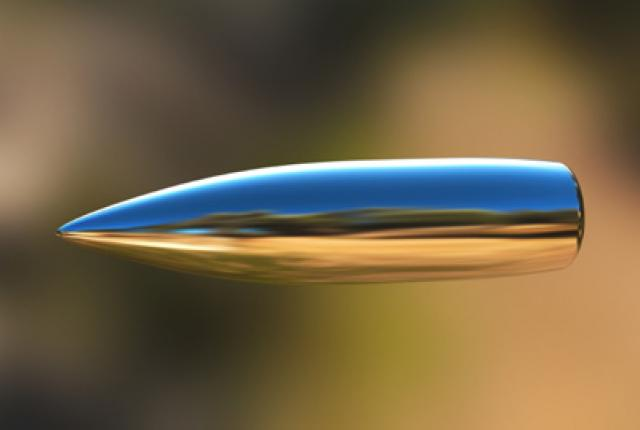The momentum of a bullet of mass 20 g fired from a gun is $10 \text{ kg m/s}$ The kinetic energy of this bullet expressed in kJ will be:

×